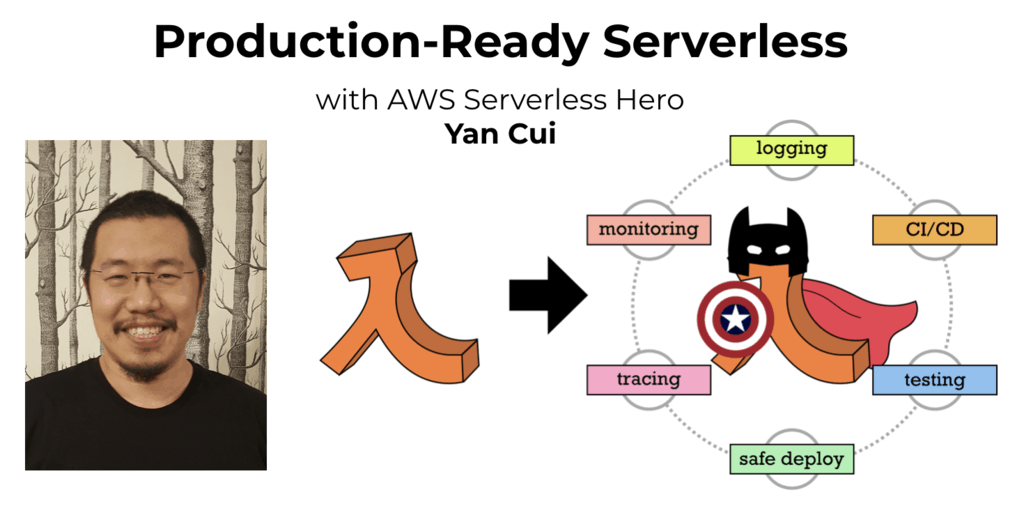# Project Euler – Problem 47 Solution

Check out my new course Learn you some Lambda best practice for great good! and learn the best practices for performance, cost, security, resilience, observability and scalability.

#### Problem

The first two consecutive numbers to have two distinct prime factors are:

14 = 2 x 7

15 = 3 x 5

The first three consecutive numbers to have three distinct prime factors are:

644 = 2² x 7 x 23

645 = 3 x 5 x 43

646 = 2 x 17 x 19

Find the first four consecutive integers to have four distinct primes factors. What is the first of these numbers?

#### Solution

```let hasDivisor(n) =
let upperBound = bigint(sqrt(double(n)))
[2I..upperBound] |> Seq.exists (fun x -> n % x = 0I)

let isPrime(n) = if n = 1I then false else not(hasDivisor(n))

let naturalNumbers = Seq.unfold (fun state -> Some(state, state+1I)) 1I

// define the sequence of prime numbers
let primeSeq = naturalNumbers |> Seq.filter isPrime |> Seq.cache

// recursive function to find the prime denominators for a number n
let rec getPrimeFactors denominators n =
if n = 1I then denominators
else
let denominator = primeSeq |> Seq.filter (fun x -> n % x = 0I) |> Seq.head
getPrimeFactors (denominators @ [denominator]) (n/denominator)

let primeFactors = getPrimeFactors []

// function to get the number of distinct prime factors a number has
let distinctPrimeFactorsCount n = primeFactors n |> Seq.distinct |> Seq.length

// define the sequence of numbers with exactly 4 distinct prime factors
let seq = naturalNumbers |> Seq.filter (fun n -> distinctPrimeFactorsCount n = 4) |> Seq.cache

seq
|> Seq.windowed 4
|> Seq.filter (fun l -> Seq.max (l) - Seq.min (l) = 3I)
```

Whilst on the surface this isn’t a very difficult problem to solve, the biggest challenge I had was in making sure the solution runs in a reasonable time hence note the various places where I used Seq.cache to help improve the performance of this code.Hi, I’m Yan. I’m an AWS Serverless Hero and the author of Production-Ready Serverless.

I specialise in rapidly transitioning teams to serverless and building production-ready services on AWS.

Are you struggling with serverless or need guidance on best practices? Do you want someone to review your architecture and help you avoid costly mistakes down the line? Whatever the case, I’m here to help.Check out my new course, Learn you some Lambda best practice for great good! In this course, you will learn best practices for working with AWS Lambda in terms of performance, cost, security, scalability, resilience and observability. Enrol now and enjoy a special preorder price of £9.99 (~\$13).Are you working with Serverless and looking for expert training to level-up your skills? Or are you looking for a solid foundation to start from? Look no further, register for my Production-Ready Serverless workshop to learn how to build production-grade Serverless applications!